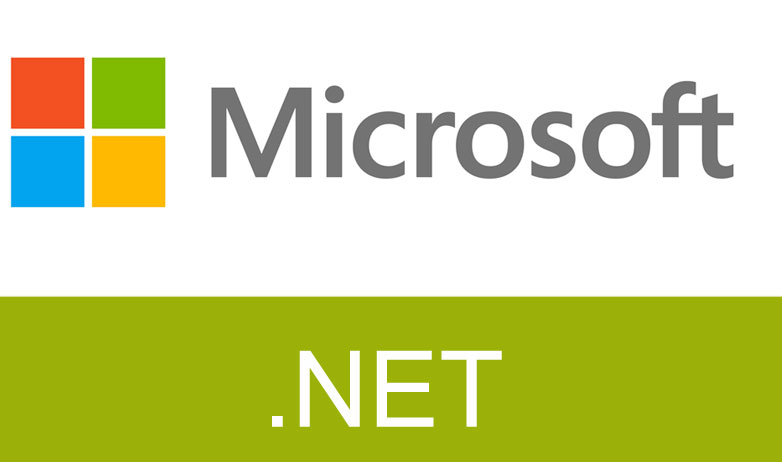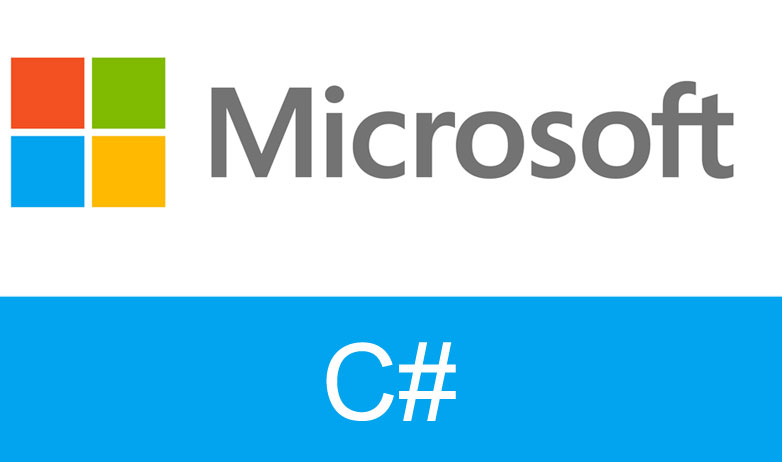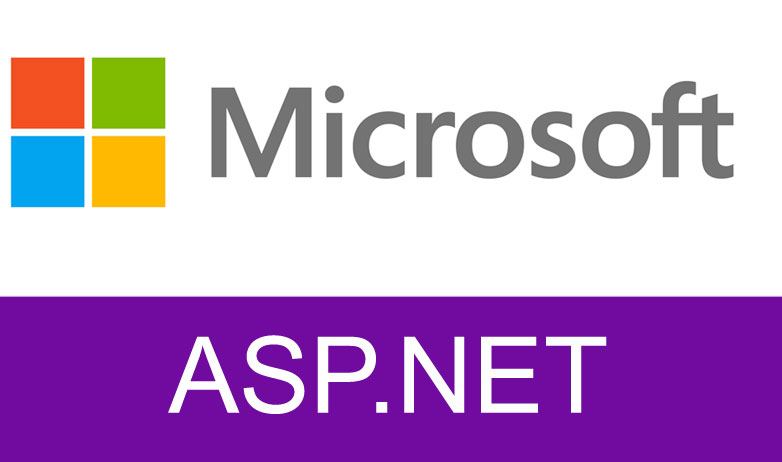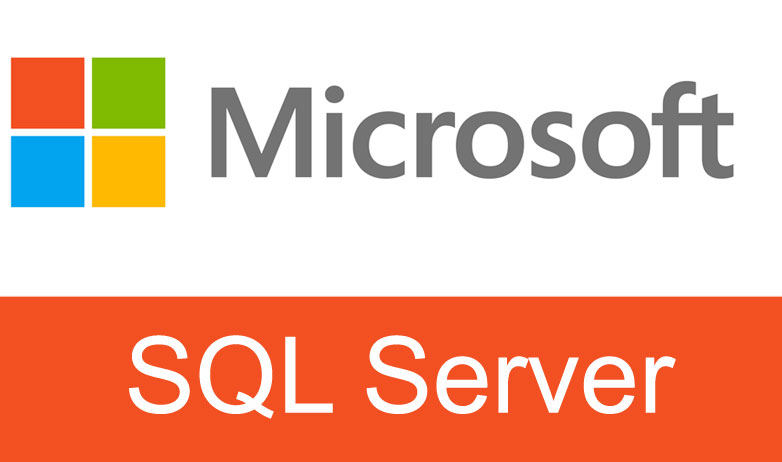1. Back To Blog

# .NET interview questions: - State the use Trim function in C#?

Trim: - The Trim function helps us to remove OFF spaces characters from the beginning and from the end of the instance.

The following are the different variation's provided by the Trim function.

1. Trim () = It simply removes OFF Spaces from both the Start and End of the instance or string.
2. TrimStart () = It simply removes OFF Spaces from the Start of the instance or string.
3. TrimEnd () = It simply removes OFF Spaces from the End of the instance or string.

Let's see a small and simple example to understand the concept of the above mentioned Trim function with their respective variations.

In order to see it practically just follow the following steps.

Step1: - Create a Console Application for that just Open Visual Studio >> go to >> File >> New >> Project >> Windows >> Select Console Application.Step2: - Now, let take the above variation one by one and demonstrate.

1. Trim () = It simply removes OFF Spaces from both the Start and End of the instance or string.

Now just simply add the below code snippet to your program.cs file.

`static void Main(string[] args)// Created a Simple String Variable with it's Value.string strMyData = " Performing the Trim Function example. ";// Call Trim instance method.// This returns a new string copy.strMyData = strMyData.Trim();// Added a Bracket So that we can see the exact performance of Trim.Console.WriteLine("["+strMyData+"]");// The below line of code will help us to see the output window.Console.ReadLine();}`

In the above code snippet you have noticed that the string variable strMyData contains value with some OFF Spaces at the start and at the end of the data.

Now, let simply run the Console application and will see the result likebelow diagram.In the above output you can clearly see that now the OFF spaces are removed from the start and end of the string.

Step3: - Similarly let's take the second variation and see it practically.

2. TrimStart () = It simply removes OFF Spaces from the Start of the instance or string.

Now just simply add the below code snippet to your program.cs file.

static void Main(string[] args)

// Created a Simple String Variable with it's Value.
string strMyData = " Performing the Trim Function example. ";
// Call TrimStart instance method.
// This returns a new string copy.
strMyData = strMyData.TrimStart();
// Added a Bracket So that we can see the exact performance of Trim.
Console.WriteLine("["+strMyData+"]");
// The below line of code will help us to see the output window.
}

Now, let simply run the Console application and will see the result like below diagram.In the above output diagram you can clearly see that the OFF Spaces are removed from the starting of the string but the end of theOFF Spaces are still not removed which means that TrimStart() function only removes the OFF spaces from the beginning of the string.

Step4: - Now, let's take the final variation provided by the Trim function and demonstrate it practically.

3. TrimEnd () = It simply removes OFF Spaces from the End of the instance or string.

Now just simply add the below code snippet to your program.cs file.

`static void Main(string[] args)// Created a Simple String Variable with it's Value.string strMyData = " Performing the Trim Function example. ";// Call TrimEnd instance method.// This returns a new string copy.strMyData = strMyData.TrimEnd();// Added a Bracket So that we can see the exact performance of Trim.Console.WriteLine("["+strMyData+"]");// The below line of code will help us to see the output window.Console.ReadLine();}`

Now, let simply run the Console application and will see the result like below diagram.Now, from the output diagram you can clearly see that the OFF spaces from the end of the string are removed while the OFF Spaces from the start are not removed, which means that the TrimEnd() basically removes only the OFF Spaces from the end of the string.

This is how exactly Trim function help us to remove OFF spaces from the string.

Go through this video on Params keyword used in C#

See for more stuffs on important Dotnet interview questions and on author's blog for Most asked .NET interview questions.

Do not forget to watch below C#(CSharp) interview questions and answers series :-Visit us @ www.questpond.com or call us at 022-66752917... read more#### .NET interview questions 6th edition (Sixth edition) - By Shivprasad Koirala#### C# Design pattern interview questions with answers: - How Singleton is different from Static class?#### Exception Handling in C# using example step by step#### MVC vs ASP.NET ( MVC Interview question with answers )?#### What is the difference between each version of MVC 2, 3, 4, 5 and 6? (MVC Interview Questions)